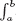# INDEX

S-I-R model

SARS, 456

threshold value, 456

Δ, Delta, notation, 9

∫, 297, 250, 255

λ, Lagrange multiplier, 390

Σ, Sigma notation, 246

d/dx notation, 103

dy/dt = ky, 424

e, 42, 495

f′ (x) notation, 98

dy/dt = k(y − A), 431

acceleration, 106

age distribution in US, 332

air pressure, 169

ampicillin, 225, 474, 477

amplitude, 72

analytical solution, 458

ancestors, 481

annuity, 470

present value, 470

antiderivative, 292

constant multiples, 299

finding formula for, 297

graphical, 292

numerical, 292

of 1/x, 300

of ekx, 300

of xn, 298

of constant, 298

of cos (kx), 300

of cos x, 300

of sin (kx), 300

of sin x, 300

properties of, 299

sums, 299

apple orchard yield, 329

approximation, see estimation

area

and definite integral, 255

between two curves, 257

asymptote, 68

of exponential decay function, 40

average cost, 202, 204

definition of, 202

marginal cost and, 204

minimizing, 204

not marginal cost, 202

visualizing, 202

average rate of change, 16

average value of function, 272

visualizing on a graph, 272

average velocity, 20

baby boom, 217

Barbra Streisand, 479

base

of exponential, 39

of natural logarithm, 46

Bay of Fundy, 164

bell-shaped curve, 346

best fit line, 486

bicycles, 23

bioavailability, 262, 323

biodiesel fuel, 27, 45